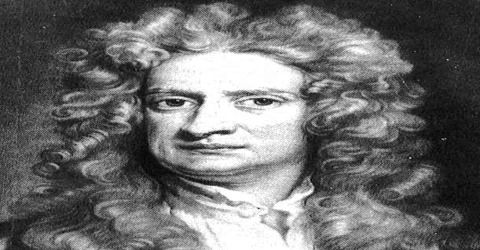# Contribution of Sir Isaac Newton in Modern Science

Contribution of Sir Isaac Newton in Successive Development of Modern Science

Newton (1642-1727): Why does a body fall on the ground? People had also interest about sun, moon, planets, stars etc in the universe since ancient time. Until seventeenth century they had idea that falling of a body to the ground is a natural phenomenon. He first expressed his opinion about the movement of the heavenly bodies. Besides his work on universal gravitation (gravity), Newton developed the three laws of motion which form the basic principles of modern physics

In the second century, Greek astronomer Tolemy put forward the ‘Geo-centric’ theory. According to this theory the sum the moon, planets, stars are revolving around the earth. In the fifteenth century astronomer Copernicus gave the ‘sun-centric’ theory. In this theory sun has been considered to be at the centre of the universe and other planets revolve around the sun. Copernicus had the idea that magnetic force compelled the planets to rotate around the sun. In the fifteenth century Kepler, by analyzing the data and information about the motion of planets and stars, came to the conclusion that the paths of the planets are not circular, rather elliptical.

He discovered some rules in the motion of planets and he expressed these rules by three laws. These are known as Kepler’s laws of planetary motion. But Kepler could not match his elliptical hypothesis with the concept of magnetic force hypothesis of Copernicus. He could not give any satisfactory explanation of the rotation of the planets around the sun. Besides, explanation of a body falling on the ground is not found in Kepler’s law. Answer of all these questions are found when Sir Isaac Newton’s famous book “Philosophiae Naturalis Principia Mathematics” was published in 1687. In this book he explained how the solid masses move and presented his theory with mathematical analysis. Besides, he presented gravitational law. He explained; the reason for the rotation of the moon around the earth in elliptical orbit and also the rotation of the planets around the sun in elliptical orbit is also due to this gravitation.

Newton’s contributions in optics and mathematics also are tremendous. He invented calculus. His discovery of calculus led the way to more powerful methods of solving mathematical problems.

Sir Isaac Newton’s Contributions in Summary:

• Newton’s three laws of motion laid the foundation of classical mechanics
• Isaac Newton invented calculus
• He was the first to formulate the notion of gravity as a universal force
• Newton’s Principia is one of the most important works in the history of science
• He discovered the generalized binomial theorem in 1665
• He invented the first reflecting telescope
• He did ground breaking work in optics
• He identified light as the source of color sensation
• He inferred correctly the oblateness of Earth’s spheroidal figure